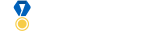Average reputation gained from questions compared to answers

0

(click on this box to dismiss)

Average reputation from questions compared to answers fro our top users, not taking into account daily rep cap at 200Q&A for programming puzzle enthusiasts and code golfers

with x as
(
select OwnerUserId, PostTypeId, count(*) as Count,
sum(Score) as Score, sum(RepCount) as RepCount, avg(RepCount) as AvgRep,
from (
case when P.PostTypeID = 1 then QRepCount else ARepCount end as RepCount
from Posts as P
left join (
select v.PostId,
Sum(case when v.VoteTypeId = 2 then 1 end) as UpVotes,
Sum(case when v.VoteTypeId = 3 then -1 end) as DownVotes,
IsNull(Sum(case when v.VoteTypeId = 2 then 5 end),0) +
IsNull(Sum(case when v.VoteTypeId = 3 then -2 end),0) as QRepCount,
IsNull(Sum(case when v.VoteTypeId = 2 then 10 end),0) +
IsNull(Sum(case when v.VoteTypeId = 3 then -2 end),0) as ARepCount
where v.VoteTypeId in (2,3)
group by v.PostId
) as V on v.PostId = P.Id
where P.PostTypeId in (1,2)
) as p
group by OwnerUserId, PostTypeId
)
select top 100
id, DisplayName,
case when q.AvgRep<a.AvgRep then 'Answers' else 'Questions' end
as More_Valued_For,
q.Count as QuestionCount, q.AvgRep as Que_AverageRep,
a.Count as AnswerCount, a.AvgRep as Ans_AverageRep
from Users U
left join x as q on u.id = q.OwnerUserId and q.PostTypeId = 1
left join x as a on u.id = a.OwnerUserId and a.PostTypeId = 2
order by a.RepCount+q.RepCount desc

Enter Parameters

Options:
-Hold tight while we fetch your results
:records returned in :time ms:cached